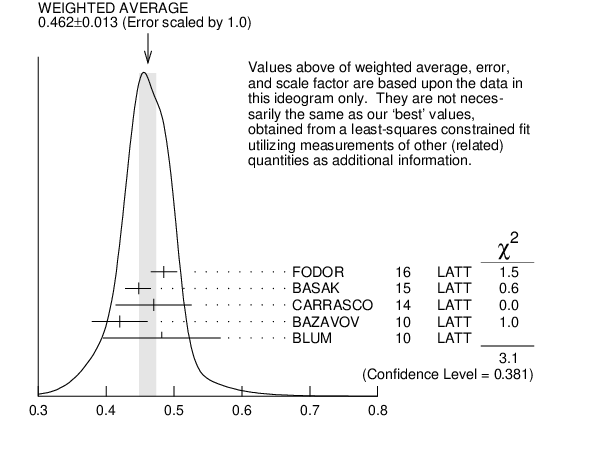# ${\boldsymbol m}_{{{\boldsymbol u}}}/{\boldsymbol m}_{{{\boldsymbol d}}}$ MASS RATIO INSPIRE search

VALUE DOCUMENT ID TECN  COMMENT
$\bf{ 0.47 {}^{+0.06}_{-0.07}}$ OUR EVALUATION
$0.485$ $\pm0.011$ $\pm0.016$ 1
 2016
LATT
$0.4482$ ${}^{+0.0173}_{-0.0206}$ 2
 2015
LATT
$0.470$ $\pm0.056$ 3
 2014
LATT
$0.698$ $\pm0.051$ 4
 2012
LATT
$0.42$ $\pm0.01$ $\pm0.04$ 5
 2010
LATT
$0.4818$ $\pm0.0096$ $\pm0.0860$ 6
 2010
LATT
• • • We do not use the following data for averages, fits, limits, etc. • • •
$0.550$ $\pm0.031$ 7
 2007
LATT
$0.43$ $\pm0.08$ 8
 2004 A
LATT
$0.410$ $\pm0.036$ 9
 2003
LATT
$0.553$ $\pm0.043$ 10
 1996
THEO Compilation
1  FODOR 2016 is a lattice simulation with ${{\mathit N}_{{f}}}$ = 2 + 1 dynamical flavors and includes partially quenched QED effects.
2  BASAK 2015 is a lattice computation using 2+1 dynamical quark flavors.
3  CARRASCO 2014 is a lattice QCD computation of light quark masses using 2 + 1 + 1 dynamical quarks, with ${{\mathit m}_{{u}}}$ = ${{\mathit m}_{{d}}}{}\not=$ ${{\mathit m}_{{s}}}{}\not=$ ${{\mathit m}_{{c}}}$. The ${\mathit {\mathit u}}$ and ${\mathit {\mathit d}}$ quark masses are obtained separately by using the ${{\mathit K}}$ meson mass splittings and lattice results for the electromagnetic contributions.
4  AOKI 2012 is a lattice computation using 1 + 1 + 1 dynamical quark flavors.
5  BAZAVOV 2010 is a lattice computation using 2+1 dynamical quark flavors.
6  BLUM 2010 is a lattice computation using 2+1 dynamical quark flavors.
7  BLUM 2007 determine quark masses from the pseudoscalar meson masses using a QED plus QCD lattice computation with two dynamical quark flavors.
8  AUBIN 2004A perform three flavor dynamical lattice calculation of pseudoscalar meson masses, with continuum estimate of electromagnetic effects in the kaon masses.
9  NELSON 2003 computes coefficients in the order $\mathit p{}^{4}$ chiral Lagrangian using a lattice calculation with three dynamical flavors. The ratio ${\mathit m}_{{{\mathit u}}}/{\mathit m}_{{{\mathit d}}}$ is obtained by combining this with the chiral perturbation theory computation of the meson masses to order $\mathit p{}^{4}$.
10  LEUTWYLER 1996 uses a combined fit to ${{\mathit \eta}}$ $\rightarrow$ 3 ${{\mathit \pi}}$ and ${{\mathit \psi}^{\,'}}$ $\rightarrow$ ${{\mathit J / \psi}}$ (${{\mathit \pi}},{{\mathit \eta}}$) decay rates, and the electromagnetic mass differences of the ${{\mathit \pi}}$ and ${{\mathit K}}$.${\mathit m}_{{{\mathit u}}}/{\mathit m}_{{{\mathit d}}}$ MASS RATIO
References:
 FODOR 2016
PRL 117 082001 Up and Down Quark Masses and Corrections to Dashen's Theorem from Lattice QCD and Quenched QED
 BASAK 2015
JPCS 640 012052 Electromagnetic Effects on the Light Hadron Spectrum
 CARRASCO 2014
NP B887 19 Up, Down, Strange and Charm Quark Masses with $\mathit N_{f}$ = 2+1+1 Twisted Mass Lattice QCD
 AOKI 2012
PR D86 034507 1+1+1 Flavor QCD + QED Simulation at the Physical Point
 BAZAVOV 2010
RMP 82 1349 Full Nonperturbative QCD Simulations with 2+1 Flavors of Improved Staggered Quarks
 BLUM 2010
PR D82 094508 Electromagnetic Mass Splittings of the Low Lying Hadrons and Quark Masses from 2+1 Flavor Lattice QCD+QED
 BLUM 2007
PR D76 114508 Determination of Light Quark Masses from the Electromagnetic Splitting of Pseudoscalar Meson Masses Computed with Two Flavors of Domain Wall Fermions
 AUBIN 2004A
PR D70 114501 Light Pseudoscalar Decay Constants, Quark Masses, and Low Energy Constants from three-flavor Lattice QCD
 NELSON 2003
PRL 90 021601 Up Quark Mass in Lattice QCD with Three Light Dynamical Quarks and Implications for Strong $\mathit CP$ Invariance
 LEUTWYLER 1996
PL B378 313 The Ratios of the Light Quark Masses